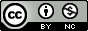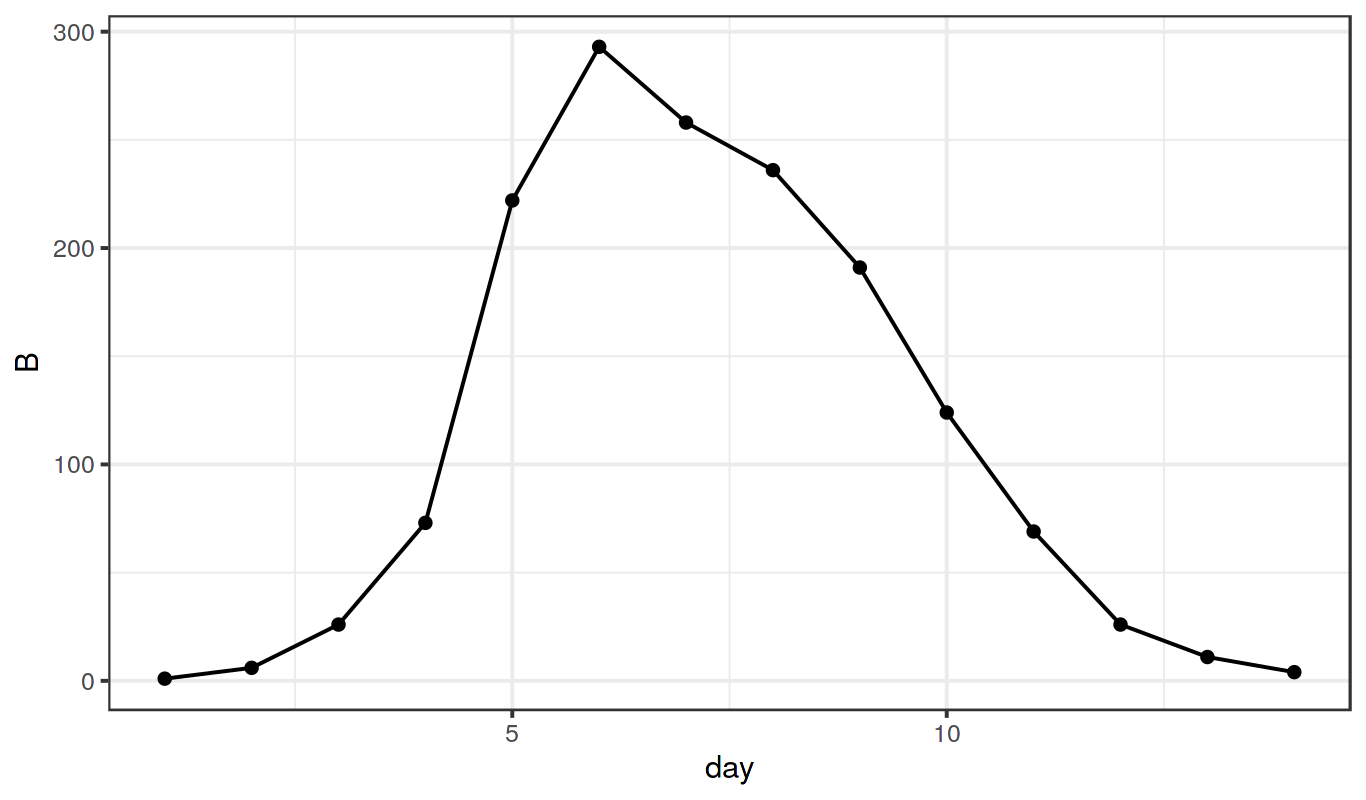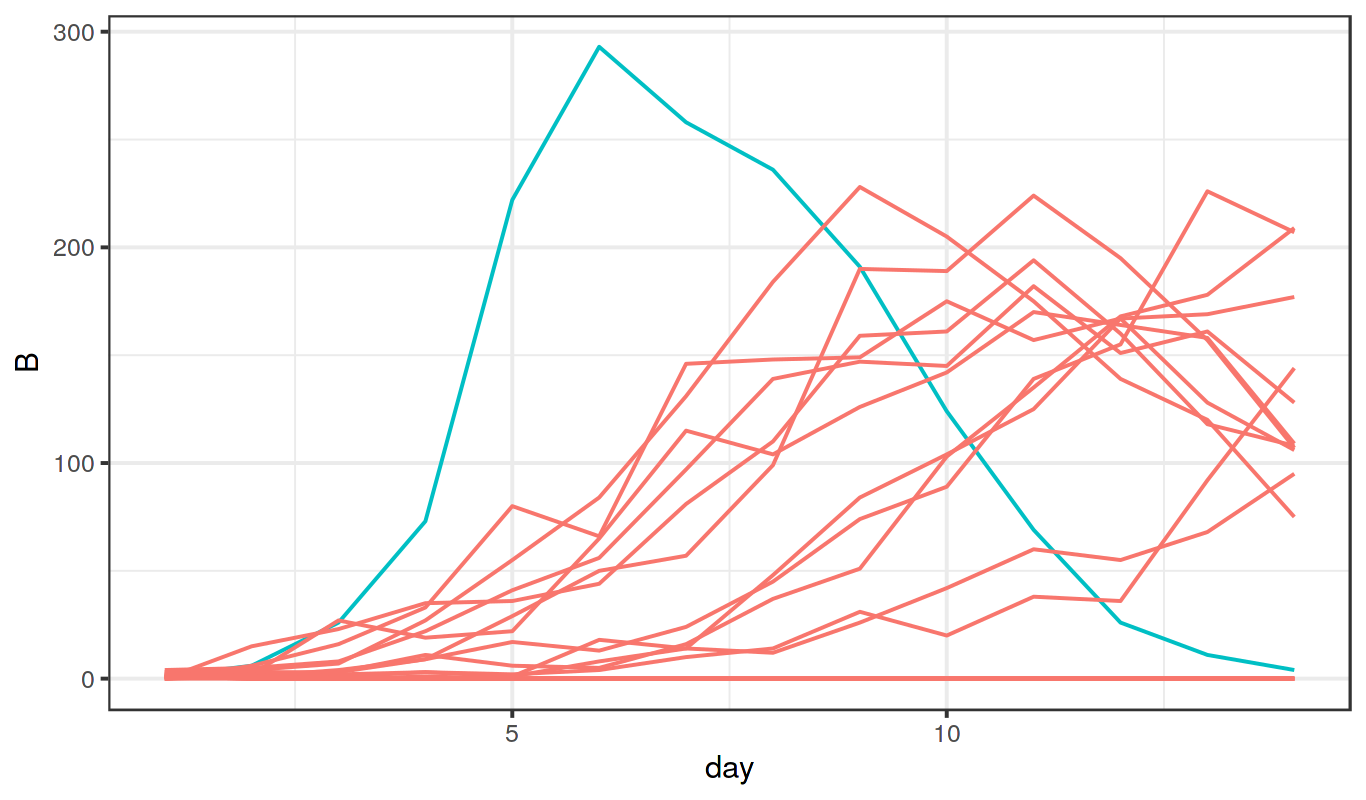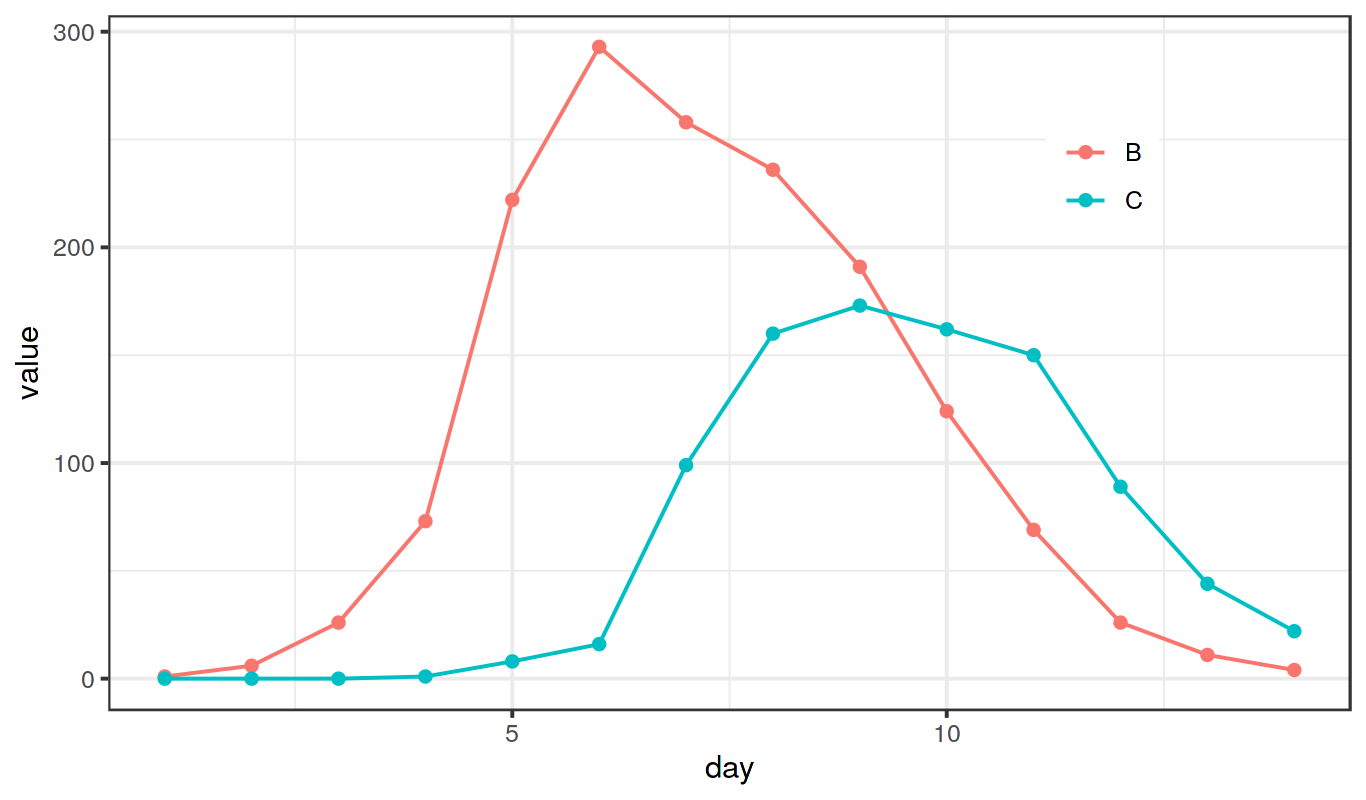Licensed under the Creative Commons Attribution-NonCommercial license. Please share and remix noncommercially, mentioning its origin.This document has its origins in the SISMID short course on Simulation-based Inference given by Aaron King and Edward Ionides.

Produced with R version 3.6.3 and pomp version 2.8.

Important Note: These materials have been updated for use with version 2.8. As of version 2, pomp syntax has changed substantially. These changes are documented on the pomp website.

## Objectives

This tutorial develops some classes of dynamic models of relevance in biological systems, especially epidemiology. We have the following goals:

1. Dynamic systems can often be represented in terms of flows between compartments. We will develop the concept of a compartment model for which we specify rates for the flows between compartments.
2. We show how deterministic and stochastic versions of a compartment model are derived and related.
3. We introduce Euler’s method to simulate from dynamic models, and we apply it to both deterministic and stochastic compartment models.

## Introduction

Compartmental models are of great utility in many disciplines and very much so in epidemiology. Let us derive deterministic and stochastic versions of the susceptible-infected-recovered (SIR) model of disease transmission dynamics in a closed population. In so doing, we will use notation that generalizes to more complex systems (Bretó et al. 2009).Diagram of the SIR compartmental model.

• Let $$S$$, $$I$$, and $$R$$ represent, respectively, the number of susceptible hosts, the number of infected (and, by assumption, infectious) hosts, and the number of recovered or removed hosts.
• We suppose that each arrow has an associated per capita rate, so here there is a rate $$\mu_{SI}$$ at which individuals in $$S$$ transition to $$I$$, and $$\mu_{IR}$$ at which individuals in $$I$$ transition to $$R$$.
• To account for demography (birth/death/migration) we allow the possibility of a source and sink compartment, which is not represented on the flow diagram above.
• We write $$\mu_{{\small\bullet} S}$$ for a rate of births into $$S$$.
• Mortality rates are denoted by $$\mu_{S{\small\bullet}}$$, $$\mu_{I{\small\bullet}}$$, $$\mu_{R{\small\bullet}}$$.
• The rates may be either constant or varying. In particular, for a simple SIR model, the recovery rate $$\mu_{IR}$$ is a constant but the infection rate has the time-varying form $\mu_{SI}(t)=\beta\,\frac{I(t)}{N(t)},$ with $$\beta$$ being the contact rate and $$N$$ the total size of the host population. In the present case, since the population is closed, we set $\mu_{{\small\bullet} S}=\mu_{S{\small\bullet}}=\mu_{I{\small\bullet}}=\mu_{R{\small\bullet}}=0.$
• In general, it turns out to be convenient to keep track of the flows between compartments as well as the number of individuals in each compartment. Let $$N_{SI}(t)$$ count the number of individuals who have transitioned from $$S$$ to $$I$$ by time $$t$$. We say that $$N_{SI}(t)$$ is a counting process. A similarly constructed process $$N_{IR}(t)$$ counts individuals transitioning from $$I$$ to $$R$$. To include demography, we could keep track of birth and death events by the counting processes $$N_{{\small\bullet} S}(t)$$, $$N_{S{\small\bullet}}(t)$$, $$N_{I{\small\bullet}}(t)$$, $$N_{R{\small\bullet}}(t)$$.
• For discrete population compartment models, the flow counting processes are non-decreasing and integer valued.
• For continuous population compartment models, the flow counting processes are non-decreasing and real valued.
• The number of hosts in each compartment can be computed via these counting processes. Ignoring demography, we have: \begin{aligned} S(t) &= S(0) - N_{SI}(t)\\ I(t) &= I(0) + N_{SI}(t) - N_{IR}(t)\\ R(t) &= R(0) + N_{IR}(t) \end{aligned} These equations represent a kind of conservation law.
• Over any finite time interval $$[t,t+\delta)$$, we have \begin{aligned} {\Delta}{S} &= -{\Delta}{N}_{SI}\\ {\Delta}{I} &= {\Delta}{N}_{SI}-{\Delta}{N}_{IR}\\ {\Delta}{R} &= {\Delta}{N}_{IR}, \end{aligned} where the $$\Delta$$ notation indicates the increment in the corresponding process. Thus, for example $${\Delta}{N}_{SI}(t) = N_{SI}(t+\delta)-N_{SI}(t)$$.

## Compartmental models in theory

### The deterministic version of the SIR model

Together with initial conditions specifying $$S(0)$$, $$I(0)$$ and $$R(0)$$, we just need to write down ordinary differential equations (ODE) for the flow counting processes. These are, $\begin{gathered} \frac{dN_{SI}}{dt} = \mu_{SI}(t)\,S(t), \qquad \frac{dN_{IR}}{dt} = \mu_{IR}\,I(t). \end{gathered}$

### The simple continuous-time Markov chain version of the SIR model

• Continuous-time Markov chains are the basic tool for building discrete population epidemic models.
• Recall that a Markov chain is a discrete-valued stochastic process with the Markov property: the future evolution of the process depends only on the current state.
• Surprisingly many models have this Markov property. If all important variables are included in the state of the system, then the Markov property appears automatically.
• The Markov property lets us specify a model by giving the transition probabilities on small intervals together with initial conditions. For the SIR model in a closed population, we have \begin{aligned} &\mathbb{P}\left[{N_{SI}(t+\delta)=N_{SI}(t)+1}\right] &=& &\mu_{SI}(t)\,S(t)\,\delta + o(\delta)\\ &\mathbb{P}\left[{N_{SI}(t+\delta)=N_{SI}(t)}\right] &=& &1-\mu_{SI}(t)\,S(t)\,\delta + o(\delta)\\ &\mathbb{P}\left[{N_{IR}(t+\delta)=N_{IR}(t)+1}\right] &=& &\mu_{IR}(t)\,I(t)\,\delta + o(\delta)\\ &\mathbb{P}\left[{N_{IR}(t+\delta)=N_{IR}(t)}\right] &=& &1-\mu_{IR}(t)\,I(t)\,\delta + o(\delta)\\ \end{aligned}
• A simple counting process is one for which no more than one event can occur at a time (Wikipedia: point process). Thus, in a technical sense, the SIR Markov chain model we have written is simple. One may want to model the extra randomness resulting from multiple simultaneous events: someone sneezing in a crowded bus, large gatherings at football matches, etc. This extra randomness may even be critical to match the variability in data.
• We will see later, in the measles case study, a situation where this extra randomness plays an important role. The representation of the model in terms of counting processes turns out to be useful for this.

##### Exercise: From Markov chain to ODE

Find the expected value of $$N_{SI}(t+\delta)-N_{SI}(t)$$ and $$N_{IR}(t+\delta)-N_{IR}(t)$$ given the current state, $$S(t)$$, $$I(t)$$ and $$R(t)$$. Take the limit as $$\delta\to 0$$ and show that this gives the ODE model.

### Euler’s method for ODE

• Euler took the following approach to numeric solution of an ODE:
• He wanted to investigate an ODE $\frac{dx}{dt}=h(x,t)$ with an initial condition $$x(0)$$. He supposed this ODE has some true solution $$x(t)$$ which could not be worked out analytically. He therefore wished to approximate $$x(t)$$ numerically.
• He initialized the numerical solution at the known starting value, $\tilde x(0)=x(0).$ Then, for $$k=1,2,\dots$$, he supposed that the gradient $$dx/dt$$ is approximately constant over the small time interval $$k\delta\le t\le (k+1)\delta$$. Therefore, he defined $\tilde{x}\big((k+1)\delta\big) = \tilde{x}(k\delta) + \delta\,h\big(\tilde{x}(k\delta),k\delta\big).$
• This defines $$\tilde x(t)$$ when only for those $$t$$ that are multiples of $$\delta$$, but let’s suppose $$\tilde x(t)$$ is constant between these discrete times.
• We now have a numerical scheme, stepping forwards in time increments of size $$\delta$$, that can be readily evaluated by computer.
• Mathematical analysis of Euler’s method says that, as long as the function $$h(x)$$ is not too exotic, then $$x(t)$$ is well approximated by $$\tilde x(t)$$ when the discretization time-step, $$\delta$$, is sufficiently small.
• Euler’s method is not the only numerical scheme to solve ODEs. More advanced schemes have better convergence properties, meaning that the numerical approximation is closer to $$x(t)$$. However, there are 3 reasons we choose to lean heavily on Euler’s method:
1. Euler’s method is the simplest (the KISS principle).
2. Euler’s method extends naturally to stochastic models, both continuous-time Markov chains models and stochastic differential equation (SDE) models.
3. In the context of data analysis, close approximation of the numerical solutions to a continuous-time model is less important than may be supposed, a topic worth further discussion….

### Some comments on using continuous-time models and discretized approximations

• In some physical situations, a system follows an ODE model closely. For example, Newton’s laws provide a very good approximation to the motions of celestial bodies.
• In many biological situations, ODE models become good approximations to reality only at relatively large scales. On small temporal scales, models cannot usually capture the full scope of biological variation and biological complexity.
• If we are going to expect substantial error in using $$x(t)$$ to model a biological system, maybe the numerical solution $$\tilde x(t)$$ represents the system being modeled as well as $$x(t)$$ does.
• If our model fitting, model investigation, and final conclusions are all based on our numerical solution $$\tilde x(t)$$ (e.g., we are sticking entirely to simulation-based methods) then we are most immediately concerned with how well $$\tilde x(t)$$ describes the system of interest.
$$\tilde x(t)$$ becomes more important than the original model, $$x(t)$$.
• When following this perspective, it is important that one fully describe the numerical model $$\tilde x(t)$$. From this point of view, then, the main advantage of the continuous-time model $$x(t)$$ is then that it gives a succinct way to describe how $$\tilde x(t)$$ was constructed.
• All numerical methods are, ultimately, discretizations. Epidemiologically, setting $$\delta$$ to be a day, or an hour, can be quite different from setting $$\delta$$ to be two weeks or a month. For continuous-time modeling, we still require that $$\delta$$ is small compared to the timescale of the process being modeled, and the choice of $$\delta$$ does not play an explicit role in the interpretation of the model.
• Putting more emphasis on the scientific role of the numerical solution itself reminds you that the numerical solution has to do more than approximate a target model in some asymptotic sense: the numerical solution should be a sensible model in its own right.

### Euler’s method for a discrete SIR model

• Recall the simple continuous-time Markov chain interpretation of the SIR model without demography: \begin{aligned} \mathbb{P}\left[{N_{SI}(t+\delta)=N_{SI}(t)+1}\right] &= \mu_{SI}(t)\,S(t)\,\delta + o(\delta),\\ \mathbb{P}\left[{N_{IR}(t+\delta)=N_{IR}(t)+1}\right] &= \mu_{IR}\,I(t)\,\delta + o(\delta). \end{aligned}

• We look for a numerical solution with state variables $$\tilde S(k\delta)$$, $$\tilde I(k\delta)$$, $$\tilde R(k\delta)$$.

• The counting processes for the flows between compartments are $$\tilde N_{SI}(t)$$ and $$\tilde N_{IR}(t)$$. The counting processes are related to the numbers of individuals in the compartments by the same flow equations we had before: \begin{aligned} {\Delta}{\tilde S} &= -{\Delta}{\tilde N}_{SI}\\ {\Delta}{\tilde I} &= {\Delta}{\tilde N}_{SI}-{\Delta}{\tilde N}_{IR}\\ {\Delta}{\tilde R} &= {\Delta}{\tilde N}_{IR}, \end{aligned}

• Let’s focus $$N_{SI}(t)$$; the same methods can also be applied to $$N_{IR}(t)$$.

• Here are three stochastic Euler schemes for $$N_{SI}$$:
1. Poisson increments: ${\Delta}{\tilde N}_{SI}\;\sim\;\mathrm{Poisson}\left(\tilde \mu_{SI}(t)\,\tilde S(t)\,\delta\right),$ where $$\mathrm{Poisson}\left(\mu\right)$$ is the Poisson distribution with mean $$\mu$$ and $\tilde\mu_{SI}(t)=\beta\,\frac{\tilde I(t)}{N}.$
2. Binomial increments with linear probability: ${\Delta}{\tilde N}_{SI}\;\sim\;\mathrm{Binomial}\left(\tilde{S}(t),\tilde\mu_{SI}(t)\,\delta\right),$ where $$\mathrm{Binomial}\left(n,p\right)$$ is the binomial distribution with mean $$n\,p$$ and variance $$n\,p\,(1-p)$$.
3. $${\Delta}{\tilde{N}}_{SI}\;\sim\;\mathrm{Binomial}\left(\tilde{S}(t),1-e^{-\tilde{\mu}_{SI}(t)\,\delta}\right)$$.
• Note that these schemes agree as $$\delta\to 0$$.
• What are the advantages and disadvantages of these different schemes? Conceptually, it is simplest to think of (1) or (2). Numerically, it is usually preferable to implement (3).

### Compartmental models via stochastic differential equations (SDE)

The Euler method extends naturally to stochastic differential equations. A natural way to add stochastic variation to an ODE $$dx/dt=h(x)$$ is $\frac{dX}{dt}=h(X)+\sigma\,\frac{dB}{dt}$ where $$B(t)$$ is Brownian motion and so $$dB/dt$$ is Gaussian white noise. The so-called Euler-Maruyama approximation $$\tilde X$$ is generated by $\tilde X\big(\,(k+1)\delta\,\big) = \tilde X( k\delta) + \delta\, h\big(\, \tilde X(k\delta)\,\big) + \sigma \sqrt{\delta} \, Z_k$ where $$Z_1,Z_2,\dots$$ is a sequence of independent standard normal random variables, i.e., $$Z_k\sim\mathrm{Normal}\left(0,1\right)$$. Although SDEs are often considered an advanced topic in probability, the Euler approximation doesn’t demand much more than familiarity with the normal distribution.

##### Exercise: SDE version of the SIR model

Write down the Euler-Maruyama method for an SDE representation of the closed-population SIR model. Consider some difficulties that might arise with non-negativity constraints, and propose some practical way one might deal with that issue.

• A useful method to deal with positivity constraints is to use Gamma noise rather than Brownian noise (Bhadra et al. 2011,@He2010,@laneri10). SDEs driven by Gamma noise can be investigated by Euler solutions simply by replacing the Gaussian noise by an appropriate Gamma distribution.

### Euler’s method vs. Gillspie’s algorithm

• A widely used, exact simulation method for continuous time Markov chains is Gillspie’s algorithm (Gillespie 1977). We do not put much emphasis on Gillespie’s algorithm here. Why? When would you prefer an implementation of Gillespie’s algorithm to an Euler solution?
• Numerically, Gillespie’s algorithm is often approximated using so-called tau-leaping methods (Gillespie 2001). These are closely related to Euler’s approach. Is it reasonable to call a suitable Euler approach a tau-leaping method?

## Compartmental models in pomp.

### The boarding-school flu outbreak

As an example that we can probe in some depth, let’s look at an isolated outbreak of influenza that occurred in a boarding school for boys in England (Anonymous 1978). Download the data and examine it:

read.table("http://kingaa.github.io/short-course/stochsim/bsflu_data.txt") -> bsflu
head(bsflu)
##              B  C day
## 1978-01-22   1  0   1
## 1978-01-23   6  0   2
## 1978-01-24  26  0   3
## 1978-01-25  73  1   4
## 1978-01-26 222  8   5
## 1978-01-27 293 16   6

The variable B refers to boys confined to bed and C to boys in convalescence. Let’s restrict our attention for the moment to the B variable.### A first POMP model

Let’s assume that $$B$$ indicates the number of boys confined to bed the preceding day and that the disease follows the simple SIR model. Our tasks will be, first, to estimate the parameters of the SIR and, second, to decide whether or not the SIR model is an adequate description of these data.

Below is a diagram of the SIR model. The host population is divided into three classes according to their infection status: S, susceptible hosts; I, infected (and infectious) hosts; R, recovered and immune hosts. The rate at which individuals move from S to I is the force of infection, $$\lambda=\mu_{SI}=\beta\,I/N$$, while that at which individuals move into the R class is $$\mu_{IR}=\gamma$$.Let’s look at how we can view the SIR as a POMP model. The unobserved state variables, in this case, are the numbers of individuals, $$S$$, $$I$$, $$R$$ in the S, I, and R compartments, respectively. It’s reasonable in this case to view the population size $$N=S+I+R$$, as fixed. The numbers that actually move from one compartment to another over any particular time interval are modeled as stochastic processes. In this case, we’ll assume that the stochasticity is purely demographic, i.e., that each individual in a compartment at any given time faces the same risk of exiting the compartment.

### Implementing the model

To implement the model in pomp, the first thing we need is a stochastic simulator for the unobserved state process. We’ve seen that there are several ways of approximating the process just described for numerical purposes. An attractive option here is to model the number moving from one compartment to the next over a very short time interval as a binomial random variable. In particular, we model the number, $${\Delta}{N_{SI}}$$, moving from S to I over interval $${\Delta}{t}$$ as ${\Delta}{N_{SI}} \sim \mathrm{Binomial}\left(S,1-e^{-\lambda{\Delta}{t}}\right),$ and the number moving from I to R as ${\Delta}{N_{IR}} \sim \mathrm{Binomial}\left(I,1-e^{-\gamma{\Delta}{t}}\right).$

A Csnippet that encodes such a simulator is as follows:

sir_step <- Csnippet("
double dN_SI = rbinom(S,1-exp(-Beta*I/N*dt));
double dN_IR = rbinom(I,1-exp(-gamma*dt));
S -= dN_SI;
I += dN_SI - dN_IR;
R += dN_IR;
")

At day zero, we’ll assume that $$I=1$$ and $$R=0$$, but we don’t know how big the school is, so we treat $$N$$ as a parameter to be estimated and let $$S(0)=N-1$$. Thus an initializer Csnippet is

sir_init <- Csnippet("
S = N-1;
I = 1;
R = 0;
")

We fold these Csnippets, with the data, into a pomp object thus:

pomp(bsflu,time="day",t0=0,rprocess=euler(sir_step,delta.t=1/6),
rinit=sir_init,paramnames=c("N","Beta","gamma"),
statenames=c("S","I","R")) -> sir

Now let’s assume that the case reports, $$B$$, result from a process by which new infections result in confinement with probability $$\rho$$, which we can think of as the probability that an infection is severe enough to be noticed by the school authorities. Since confined cases have, presumably, a much lower transmission rate, let’s treat $$B$$ as being a count of the number of boys who have moved from I to R over the course of the past day. We need a variable to track this. Let’s modify our Csnippet above, adding a variable $$H$$ to track the incidence. We’ll then replace the rprocess with the new one.

sir_step <- Csnippet("
double dN_SI = rbinom(S,1-exp(-Beta*I/N*dt));
double dN_IR = rbinom(I,1-exp(-gamma*dt));
S -= dN_SI;
I += dN_SI - dN_IR;
R += dN_IR;
H += dN_IR;
")

sir_init <- Csnippet("
S = N-1;
I = 1;
R = 0;
H = 0;
")

pomp(sir,rprocess=euler(sir_step,delta.t=1/6),rinit=sir_init,
paramnames=c("Beta","gamma","N"),statenames=c("S","I","R","H")) -> sir

Now, we’ll model the data, $$B$$, as a binomial process, $B_t \sim \mathrm{Binomial}\left(H(t)-H(t-1),\rho\right).$ But we have a problem, since at time $$t$$, the variable H we’ve defined will contain $$H(t)$$, not $$H(t)-H(t-1)$$. We can overcome this by telling pomp that we want H to be set to zero immediately following each observation. We do this by setting the accumvars argument to pomp:

pomp(sir,accumvars="H") -> sir

Now, to include the observations in the model, we must write an rmeasure component:

rmeas <- Csnippet("B = rbinom(H,rho);")

and put these into our pomp object:

sir <- pomp(sir,rmeasure=rmeas,statenames="H",paramnames="rho")

### Testing the model: simulations

Let’s perform some simulations, just to verify that our codes are working as intended. To do so, we’ll need some parameters. A little thought will get us some ballpark estimates. In the data, it looks like there were a total of 1540 infections, so the population size, $$N$$, must be somewhat in excess of this number. In fact, we can use the final-size equation $R_0 = -\frac{\log{(1-f)}}{f},$ where $$f=R(\infty)/N$$ is the final size of the epidemic, together with the idea that $$R_0$$ for influenza is typically thought to be around 1.5, to estimate that $$f\approx 0.6$$, whence $$N\approx 2600$$. If the infectious period is roughly 1 da, then $$1/\gamma \approx 1~\text{da}$$ and $$\beta = \gamma\,R_0 \approx 1.5~\text{da}^{-1}$$. Let’s simulate the model at these parameters.

sims <- simulate(sir,params=c(Beta=1.5,gamma=1,rho=0.9,N=2600),
nsim=20,format="data.frame",include.data=TRUE)

ggplot(sims,mapping=aes(x=day,y=B,group=.id,color=.id=="data"))+
geom_line()+guides(color=FALSE)##### Exercise: Explore the SIR model

Fiddle with the parameters to see if you can’t find parameters for which the data are a more plausible realization.

##### Exercise: The SEIR model

Below is a diagram of the so-called SEIR model. This differs from the SIR model in that infected individuals must pass a period of latency before becoming infectious.Modify the codes above to construct a pomp object containing the flu data and an SEIR model. Perform simulations as above and adjust parameters to get a sense of whether improvement is possible by including a latent period.

##### Exercise: Rethinking the boarding-school flu data

In the preceding, we’ve been assuming that $$B_t$$ represents the number of boys sent to bed on day $$t$$. Actually, this isn’t correct at all. As described in the report (Anonymous 1978), $$B_t$$ represents the total number of boys in bed on day $$t$$. Since boys were potentially confined for more than one day, the data count each infection multiple times. On the other hand, we have information about the total number of boys at risk and the total number who were infected. In fact, we know that $$N=763$$ boys were at risk and 512 boys in total spent between 3 and 7 days away from class (either in bed or convalescent). Moreover, we have data on the number of boys, $$C_t$$, convalescent at day $$t$$. Since $$1540~\text{boy-da}/512~\text{boy} \approx 3~\text{da}$$, we know that the average duration spent in bed was 3 da and, since $$\sum_t\!C_t=924$$, we can infer that the average time spent convalescing was $$924~\text{boy-da}/512~\text{boy} \approx 1.8~\text{da}$$.Formulate a model with both confinement and convalescent stages. Implement it in pomp using a compartmental model like that diagrammed below.You will have to give some thought to just how to model the relationship between the data ($$B$$ and $$C$$) and the state variables. How many parameters can reasonably be fixed? How many must be estimated? Obtain some ballpark estimates of the parameters and simulate to see if you can plausibly explain the data as a realization of this model.